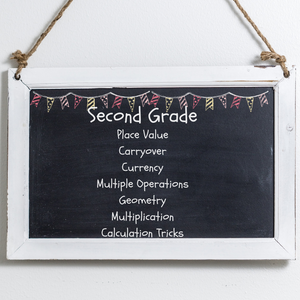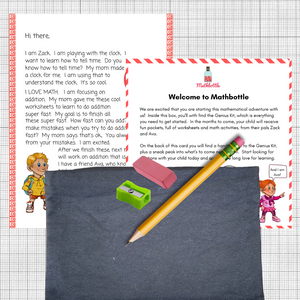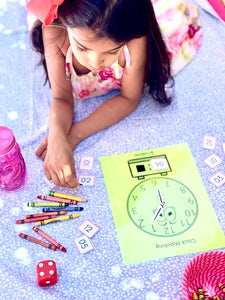# Club Mago (Age 7-8)

Regular price
\$59.95
Sale price
\$59.95
Regular price
Sold out
Unit price
per

Club Mago(Age 7-8)

Fluently add and subtract within 3 digit numbers using mental strategies.  Add up to 5 2-digit numbers using 10 frame strategies.

Understand rectangular arrays and write an equation to express the total as a sum of equal addends. Understand the parts of 3 digit numbers and their place value.

We introduce measurement, time and geometry, so that it is extremely fun to do.

Please note: The pictures are examples of the projects and activities that will be included in the first kit.  There will be similar or more fun 3-4 math activities in the months to follow.

WHAT THEY LEARN’

Operations and Algebraic Thinking

• Subtraction Strategies
• Problem Solving at various levels
• Doubles and Near Doubles
• Making Ten to Add & Subtract
• Odd and Even
• Arrays

Numbers and Operations in Base 10

• Place Value
• Skip Counting by 5s, 10s and 100s
• Expanded and Standard Form
• Base 10 Place Value
• Greater than, Less than and Equal
• Problem Solving practice
• Fact Families
• Adding and Subtracting within 1000
• Adding and Subtracting 10 or 100

Geometry

• Solid Figures
• Plane Shapes
• Rows and Columns
• Equal Parts
• Fractions

Measurement and Data

• Measuring Length
• Comparing Length